# Test: Electromagnetic Waves

## 10 Questions MCQ Test Topicwise Question Bank for Electronics Engineering | Test: Electromagnetic Waves

Description
Attempt Test: Electromagnetic Waves | 10 questions in 30 minutes | Mock test for Electrical Engineering (EE) preparation | Free important questions MCQ to study Topicwise Question Bank for Electronics Engineering for Electrical Engineering (EE) Exam | Download free PDF with solutions
QUESTION: 1

### Consider the following statements associated with an electromagnetic wave in free space: At every point in space, the electric field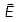and th emagnetic vector field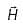are perpendicular to each other and to the direction of propagation. Velocity of propagation of wave in free space is equal to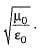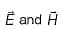oscillate in phase opposition and the ratio of their amplitude is constant being equal to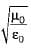Whatever may be the frequency , the electromagnetic wave travels in space with velocity of light. Which of the above statements are correct?

Solution:
• Velocity of propagation of EM-wave in free space is equal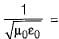= speed of light (3 x 108 m/s). Hence statement-2 is not correct.
•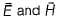oscillate in phase and the ratio of their amplitude is constant being equal to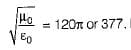. Hence, statement-3 is not correct.

Thus, statements-1 and 4 are only correct.

QUESTION: 2

### Intrinsic impedance or charcteristic impedance of the non-conducting medium in the free space is

Solution:

Intrinsic impedance or characteristic impedance of the non-conducting medium in free space is given as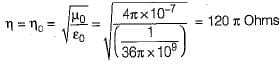QUESTION: 3

### If the magnitude of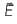in a plane wave is 1 V/m, what is the magnitude of H for a plane wave in free space?

Solution:

We know that,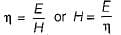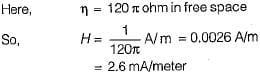QUESTION: 4

What is the velocity of a plane wave in a lossless medium having a relative permittivity of 5 and relative permeability of unity?

Solution:

Velocity of propagation is given by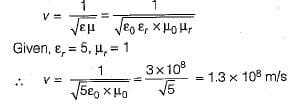QUESTION: 5

A plane wave in free space has an average Poynting vector of 1 Wm-2. The average energy density is

Solution:

Poynting vector P= EH and total energy density W= μH2.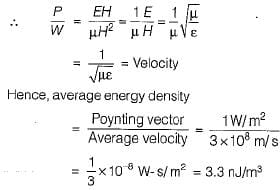QUESTION: 6

Infree space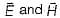are respectively given by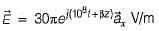and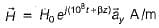The value of β and H0 are respectively

Solution: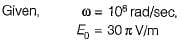This is a plane wave.
For plane wave in space,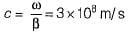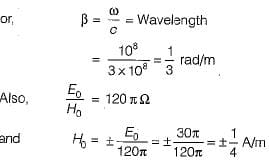QUESTION: 7

In a homogeneous non-conducting region where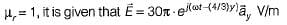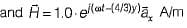The value of ∈r and ω are

Solution: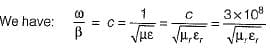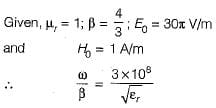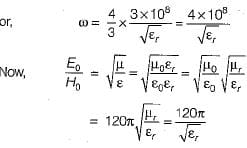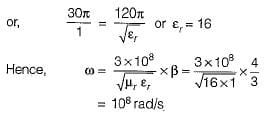QUESTION: 8

In free space it is given that,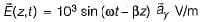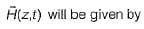Solution:

Looking at the phase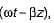we can find that direction of propagation is +z.
Since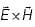must also lie in a + z direction, A must have the direction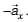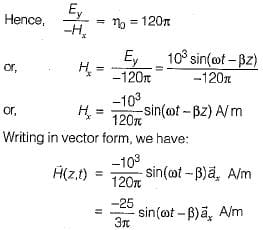QUESTION: 9

Assertion (A): Uniform plane electromagnetic waves are transverse in nature.
Reason (R): Uniform plane electromagnetic
waves have components of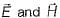only in direction perpendicular to the direction of propagation and not in the direction of propagation.

Solution:
QUESTION: 10

Assertion (A): The term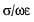is sometimes referred to the loss tangent.
Reason (R): The two vectors Jc (conduction current density) and Jd (displacement current density) are 180° out of phase and are in the opposite direction in space.

Solution: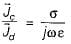which indicates that the two vectors Jc and Jd are out of phase in time and are in the same direction in the space. Hence, reason is not a correct statement.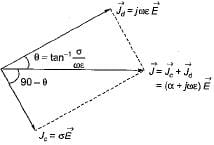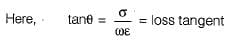Use Code STAYHOME200 and get INR 200 additional OFF Use Coupon Code